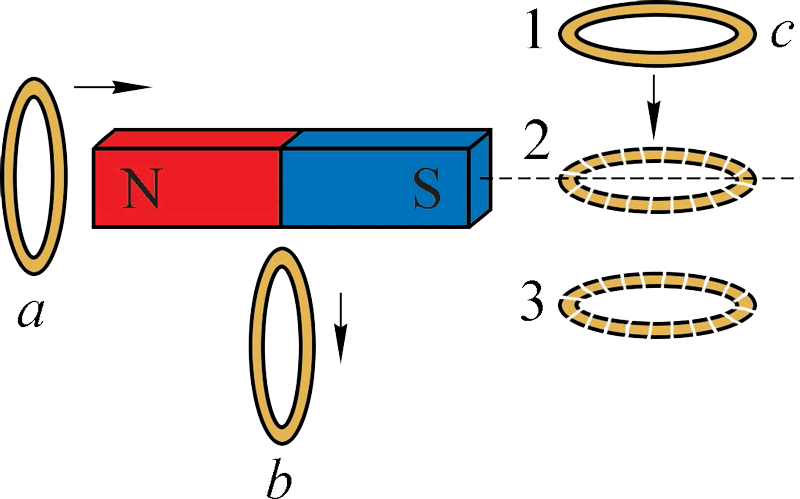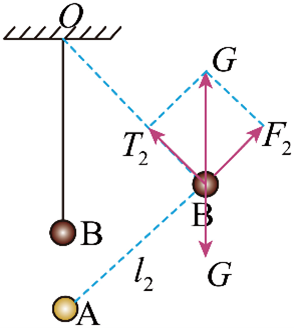## 杨浦区2022学年第一学期期末

•  2022/12/15
•  184 次
•  829 K

1．声波

（A）能在真空中传播                                  （B）在气体中的传播速度最大

（C）靠各质点的迁移来传递能量              （D）传播过程中形成疏密相间的波

【答案】

D

【解析】

A．声波不能在真空中传播，选项 A 错误；

B．声波在固体中的传播速度最大，在气体中的速度最小，选项 B 错误；

C．机械波传播过程中各质点不随波迁移，选项 C 错误；

D．声波是纵波，在传播过程中形成疏密相间的波，选项 D 正确。

2．物体处于以下状态时所受合力为零的是

（A）铅球在沙坑里下陷到最低位置时

（B）小球竖直向上抛出到速度减为零时

（C）摆球在竖直平面内摆动到右侧最高位置时

（D）小球沿竖直光滑圆轨道滑动到与圆心等高且速度为零时

【答案】

A

【解析】

A．铅球在沙坑里下陷到最低位置时，受合力为零，选项 A 正确；

B．小球竖直向上抛出到速度减为零时还受重力作用，合力不为零，选项 B 错误；

C．摆球在竖直平面内摆动到右侧最高位置时，摆球受重力和摆线的拉力作用，合力不为零，选项 C 错误；

D．小球沿竖直光滑圆轨道滑动到与圆心等高且速度为零时，小球受重力作用，合力不为零，选项 D 错误。

3．如图，足够长的固定粗糙直杆与水平面成 θ 角，套在杆上的小球在拉力F作用下以一定的初速度向上滑动。下列情况中小球机械能可能守恒的是【答案】

D

【解析】

A．F 做正功，可能还有摩擦力做负功，机械能不守恒。选项 A 不符合题意；

B．F 做正功，摩擦力做负功，机械能不守恒。选项 B 不符合题意；

C．该图中力 F 与运动方向垂直，则力 F 不做功，但是一定有摩擦力做负功，则机械能一定不守恒，选项 C 不符合题意；

D．该图中若满足 F = mgcosθ，则此时摩擦力为零，摩擦力与力 F 都不做功，则机械能守恒。选项 D 正确。

4．关于通电导线在磁场中受到的磁场力，下列说法正确的是

（A）磁场力方向可以不与磁场方向垂直

（B）磁场力的方向一定垂直于通电导线

（C）环形通电线圈在磁场中所受磁场力一定为零

（D）只有垂直磁场方向放置的通电导线才受磁场力

【答案】

B

【解析】

AB．磁场力方向一定与磁场方向垂直，一定与通电导线垂直，选项 A 错误，B 正确；

C．环形通电线圈在非匀强磁场中受磁场力不一定为零，选项 C 错误。

D．只要通电导线与磁场方向不平行就一定受磁场力，选项 D 错误。

5．若带电粒子仅在电场力作用下运动，则带电粒子可能

（A）速率变大，电势能变大              （B）所受电场力不变，动能不变

（C）速率不变，电势能不变              （D）所受电场力变小，动能不变

【答案】

C

【解析】

A．带电粒子仅在电场力作用下运动，若电场力做正功，则速率变大，动能变大，电势能变小，选项 A 错误；

B．若在匀强电场中运动，则粒子所受电场力不变，但是电场力一定做功，则动能不可能不变，选项 B 错误；

C．若带电粒子在点电荷电场中做匀速圆周运动，则速率不变，动能不变，电势能不变，选项 C 正确；

D．若带电粒子在电场中所受电场力变小，则电场力一定做功，动能不可能不变，选项 D 错误；

6．如图，空间固定一条形磁体（其轴线水平），穿过圆环的磁通量先增大再减小的是

（A）圆环 a 沿磁体轴线由磁体 N 极向右移至 S 极

（B）圆环 a 沿磁体轴线由磁体 N 极左侧向左移至无穷远

（C）圆环 b 从磁体正下方竖直下落

（D）圆环 c 从磁体右边的位置 1 下降到位置 3

【答案】

A

【解析】

A．磁体内部的磁场方向与磁体外部的磁场方向相反，圆环 a 由条形磁体的 N 极通过条形磁体移到 S 极，由于磁体内部的磁感线均穿过圆环 a，且磁体外部穿过的磁感线条数先减少后增多，故此过程磁通量先增加后减小，故 A 符合题意；

B．圆环 a 沿磁体轴线由磁体 N 极左侧向左移至无穷远，穿过圆环 a 的磁感线条数变少，通过圆环 a 的磁通量变小，故 B 不符合题意；

C．垂直于地面的圆环 b 从条形磁体的中央位置竖直下落，离磁体越远，磁场越弱，穿过圆环 b 的磁感线条数变少，磁通量随之变小，故 C 不符合题意；

D．圆环 c 从 1 到 2 过程中，磁通量减小。到 2 位置时，磁感线与圆环平行，磁通量最小为零，从 2 到 3 圆环 c 磁通量增大，故 D 不符合题意。

7．如图为“用 DIS 研究加速度与力的关系”实验的 a F 关系，根据图线分析该实验过程可能存在的问题为

（A）所用小车质量过大

（B）所挂钩码的总质量太大

（C）导轨与小车间摩擦太大

（D）没有多次测量取平均值

【答案】

B

【解析】

AB．当所挂钩码的质量远小于小车质量时，可认为钩码的重力近似等于小车的牵引力，此时 a – F 图像为过原点的倾斜的直线，若随着钩码质量的增加不满足挂钩码的质量远小于小车质量的关系时，则图像出现弯曲，即造成该图像末端弯曲的原因是所挂钩码的总质量太大，选项 A 错误，B 正确；

CD．图像在 a 轴上有截距，说明当 F = 0 时小车就有了加速度，则其原因是平衡摩擦力时木板一端抬的过高，平衡摩擦力过度，并不是导轨与小车间摩擦太大或者没有多次测量取平均值造成的，选项 CD 错误。

8．如图，A、B 两小球沿倒置的光滑圆锥内侧在水平面内做匀速圆周运动。则

（A）A 球质量大于 B 球

（B）A 球线速度大于 B 球

（C）A 球转动周期小于 B 球

（D）A 球向心加速度小于 B 球

【答案】

B

【解析】小球的受力分析如图所示，小球受到重力和支持力，它们的合力提供向心力，根据牛顿第二定律，有

$$\frac{{mg}}{{\tan \theta }}$$ = ma = m $$\frac{{{v^2}}}{r}$$

A．由表达式可知不能比较两球质量关系，选项 A 错误；

B．根据 v = $$\sqrt {\frac{{gr}}{{\tan \theta }}}$$

C．根据 T = $$\frac{{2\pi r}}{v}$$ = 2π $$\sqrt {\frac{{r\tan \theta }}{g}}$$

D．根据 a = $$\frac{{g}}{{\tan \theta }}$$ 可知 A、B 的向心加速度相等，选项 D 错误。

9．某同学在解题过程中得到 x = $$\frac{B}{E}$$ 这一表达式，其中 B 是某磁场的磁感应强度，E 是某电场的电场强度。请你帮他判断 x 的单位是

（A）kg·m             （B）s/m                （C）kg·A/s           （D）m/（A·s）

【答案】

B

【解析】

E 的单位是 N/C，B 的单位 N/(A∙m)，则 x 的单位

$$\frac{{{\rm{N/(A}} \cdot {\rm{m)}}}}{{{\rm{N/C}}}}$$ = $$\frac{{\rm{C}}}{{{\rm{A}} \cdot {\rm{m}}}}$$ = $$\frac{{{\rm{A}} \cdot {\rm{s}}}}{{{\rm{A}} \cdot {\rm{m}}}}$$ = s/m

10．如图，采用一个电阻箱和电流表测量一节干电池的电动势和内阻，下列图像中可能正确的是【答案】

A

【解析】

A．由闭合电路欧姆定律 I = $$\frac{E}{{R + r}}$$ 可知，IR 的函数为减函数、图像为非线性图像，且当 R = 0 时 I ≠ 0。选项 A 正确；

B．根据 R = $$\frac{E}{I}$$ − r，当 I = 0 时 R → ∞。选项 B 错误；

C．根据 R = $$\frac{1}{I}$$ Er 可知：R I−1 图像截距为负。选项 C 错误；

D．根据 $$\frac{1}{I}$$ = $$\frac{1}{E}$$R + $$\frac{r}{E}$$ 可知则 I−1 R 图像截距为正，选项 D 错误。

11．如图，悬挂在丝线下的小球 B 与固定在悬点正下方的小球 A 带同种电荷，两球均可视作点电荷。细线竖直时，B 处于静止状态且丝线上的拉力大小为 T1，两球间距为 l1，库仑力大小为 F1。若将 B 拉至图中另一位置处也能静止，此时丝线拉力大小为 T2，两球间距为 l2，库仑力大小为 F2。则

（A）T1 > T2                           （B）F1 < F2

（C）T2 = T1 + F1 − $$\frac{{{l_1}}}{{{l_2}}}$$ F2     （D）T2 = T1 + F1 – $$\frac{{{l_2}}}{{{l_1}}}$$ F2

【答案】

C

【解析】

B．根据库仑定律有 $$\frac{{{F_1}}}{{{F_2}}}$$ = $$\frac{{l_2^2}}{{l_1^2}}$$，由于 l1 < l2，则 F1 > F2。选项 B 错误；CD．对小球受力分析如图所示。

B 球在竖直位置时有 T1 + F1 = G

B 球在另一位置时，设细绳长为 L，由相似三角形法可得：$$\frac{G}{{L + {l_1}}}$$ = $$\frac{{{T_2}}}{{{L}}}$$ = $$\frac{{{F_2}}}{{{l_2}}}$$，由比例性质可知 $$\frac{{G - {T_2}}}{{{l_1}}}$$ 也与上式相等，即 $$\frac{{G - {T_2}}}{{{l_1}}}$$ = $$\frac{{{F_2}}}{{{l_2}}}$$ 可得：

T2 = G – $$\frac{{{l_1}}}{{{l_2}}}$$ F2 = T1 + F1 – $$\frac{{{l_1}}}{{{l_2}}}$$ F2

A．将 $$\frac{{{F_1}}}{{{F_2}}}$$ = $$\frac{{l_2^2}}{{l_1^2}}$$ 代入 F1 – $$\frac{{{l_1}}}{{{l_2}}}$$ F2，可将此式表达为 F1（1 − $$\frac{{l_1^3}}{{l_2^3}}$$），由于 l1 < l2，可证 F1 – $$\frac{{{l_1}}}{{{l_2}}}$$ F2 > 0。代入选项 C，可知 T1 < T2。选项 A 错误。

12．均匀材质制作的弹性绳沿 x 轴放置，t = 0 时刻弹性绳上处于坐标原点 O 的波源 S 沿 y 轴正向开始振动，振动周期为 0.8 s。产生的简谐横波沿弹性绳向 x 轴正、负两个方向传播，t = 1 s 时坐标 xP = 3.6 cm 的质点 P 恰好第一次处于 y 轴正向最大位移处。再过 1 s 位于 x 轴负半轴的质点 Q 恰好第一次处于 y 轴正向最大位移处。则

（A）xQ = − 7.2 cm

（B）波速为 3.6 cm/s

（C）若波源振动周期为 1 s，则 P、Q 两质点都起振后运动方向始终相反

（D）若波源振动周期为 0.4 s，则 P、Q 两质点都起振后运动方向始终相反

【答案】

D

【解析】

B．因为 t = 1 s 时坐标 xP = 3.6 cm 的质点 P 恰好第一次处于 y 轴正向最大位移处，可知质点 P 振动了 T/4 = 0.2 s，波传到 P 点用时间为 0.8 s，则波速为

v = s/t = 3.6/0.8 = 4.5 cm/s

A．再过 1 s 位于 x 轴负半轴的质点 Q 恰好第一次处于y轴正向最大位移处，可知波传到 Q 点用时间 2 s – T/4 = 1.8 s，则

xQ = − vt = −4.5×1.8 cm = − 8.1 cm

C．若波源振动周期为 1 s，则波长为 λ = vT= 4.5 cm

D．若波源振动周期为 0.4 s，则波长为 1.8 cm，x 轴正方向上关于 Q 点的对称位置 Q′ 与 P 点相距 4.5 cm 为半波长的奇数倍，则 P、Q 两质点都起振后运动方向始终相反，选项 D 正确。

13．一个物体在恒力作用下做匀加速直线运动，其速度是否可以不断增大直至无穷大？你的回答是_______（选填“是”或“否”），理由是_____________________。

【答案】

14．2022 年 11 月 12 日我国发射的天舟五号货运飞船与在轨运行的空间站组合体顺利完成自主快速交会对接。已知地球半径为 R，地面重力加速度大小为 g。若空间站组合体绕地球飞行的速度大小为 v，则对接时天舟五号货运飞船离地高度为_____________，空间站组合体的加速度大小为____________。

【答案】

$$\frac{{g{R^2}}}{{{v^2}}}$$ − R；$$\frac{{{v^4}}}{{g{R^2}}}$$

【解析】

（1）根据万有引力作为向心力：$$\frac{{GMm}}{{{r^2}}}$$ = m $$\frac{{{v^2}}}{r}$$ 可得 v = $$\sqrt {\frac{{GM}}{r}}$$

h = rR = $$\frac{{g{R^2}}}{{{v^2}}}$$  − R

（2）空间站组合体的加速度大小为 a = $$\frac{{{v^2}}}{r}$$ = $$\frac{{{v^4}}}{{g{R^2}}}$$

15．已知真空中单个点电荷在空间任一点产生的电势与该点到点电荷的距离成反比。如图，处于真空中的立方体一顶点处放置一电荷量为 + q 的点电荷。则立方体上各顶点中与 b 点电势相等的点还有______________。若在 f 点再放置一个电荷量为－q 的点电荷，则立方体上各顶点中电场强度相同的成对点有______________。

【答案】

d、f；e 与 g、b 与 d

【解析】

（1）因 b、d、f 三点到点电荷 + q 的距离相等，则立方体上各顶点中与 b 点电势相等的点还有 d、f 两点；

（2）若在 f 点再放置一个电荷量为 − q 的点电荷，则立方体上各顶点中电场强度大小和方向都相同的成对点有 g、e 两点和 b、d 两点。

16．在平直轨道上匀速行驶的观光小火车全长为 15 m，一乘客从车尾的座位上起身以 3 m/s2 的加速度匀加速跑到车头。当他抵达车头时，车头正好经过地面观察站，观察站里的安全监管员测得此人的速率为 63 km/h，于是立即叫停列车。则观光小火车的车速为___________m/s，观光小火车在此人跑动时间内走过的路程为____________m。

【答案】

8.0；25.320

【解析】

（1）v = 63 km/h = 17.5 m/s。设乘客从车尾到车头用时间 t，火车的速度 v0，由题意可知 ss = Lv0t + $$\frac{1}{2}$$ at2v0t = L，解得 t = $$\sqrt {10}$$ s

（2）观光小火车在此人跑动时间内走过的路程为 s = v0t ≈ 25.3 m/s

17．如图，电源电动势 E = 12 V，内阻 r = 1 Ω。R1R2 为两个可调电阻。已知电动机的额定电压 U = 8 V，线圈电阻 RM = 1 Ω。开关 S 闭合后，可调电阻 R1 取 1 Ω、R2 取 8 Ω 时，电动机恰能正常工作，则电动机正常工作时的效率 η = ________。在电动机正常工作的前提下调节电阻 R1R2 的取值，则电源输出功率能达到的最大值为_________W。

【答案】

87.5%；32

【解析】

（1）根据闭合电路欧姆定律，电动机正常工作时有

E = U + IR1 + Ir

12 = 8 + I(1 + 1)

I = 2 A

（2）设 R1 两端的电压为 U1，输出功率可表示为

P = UI = （U + U1）$$\frac{{E - U - {U_1}}}{r}$$ = （4 – U1）（8 + U1） = − （U1 + 2）2 + 36

U1 = 0 时，P 有最大值为 32 W

18．某同学独自一人进行“研究共点力的合成”这一实验。因无法同时拉两个弹簧测力计并记录拉力的方向和大小，该同学的实验操作步骤如下 ：

① 在木板上固定白纸，橡皮筋的一端用图钉固定在木板上 A 点，另一端系上两根带绳套的细绳，在白纸上标出某标记点“O”；

② 先将细绳 1 的绳套拉至另一标记点“B”并用图钉固定，再用弹簧测力计拉细绳 2，将橡皮筋的另一端拉至“O”点并使两细绳间有一定的夹角 θ，如图所示。记录此时弹簧测力计的示数 F1 及细绳 2 的方向（沿 OC 方向）；

③松开细绳 1 的绳套端，用弹簧测力计拉细绳 2，再次将橡皮筋的另一端拉到“O”点，记录此时弹簧测力计的示数 F2 及细绳 2 的方向（沿图中虚线方向）；

④_________________________________________，记录此时弹簧测力计的示数 F3

⑤确定标度，作出三个力的图示，观察这些力的图示间的几何关系；

⑥改变标记点“O”、“B”的位置，重复上述实验过程，多次实验。

（1）请写出在上述步骤中遗漏了什么环节：____________________________________；

（2）为完成这一实验，这位同学应在步骤④中进行的操作过程为：________________________________________________________________________________________；

（3）这位同学根据记录的三个力 F1F2F3 作图，下列图示符合这位同学的实验过程的是（4）这位同学想进一步进行合力与分力对应关系的探究，他在完成实验步骤 ①、②后，保持橡皮筋的另一端仍在“O”点并缓慢改变细绳 2 的方向，观察到弹簧测力计的读数逐渐增大，则两细绳间夹角 θ 的变化情况为_________________________。

【答案】

（1）使用弹簧测力计前需要对弹簧测力计进行调零；

（2）用弹簧测力计拉细绳 1 并将细绳 2 的绳套端拉直固定，使橡皮筋的另一端在“O”点且满足细绳 1 沿 OB 方向、细绳 2 沿 OC 方向；

（1）上述步骤中没有标记 C 点位置。

（2）将细绳 2 的绳套拉至标记点 C 并用图钉固定，再用弹簧测力计拉细绳1，将橡皮筋的另一端拉至 O 点，且使细绳 1 沿 OB 方向。

（3）D；

（4）若 θ 的初始值等于 90°，θ 可能增大也可能减小；若 θ 的初始值小于 90°，θ 一直减小；若 θ 的初始值大于 90°，θ 一直增大。

【解析】

（3）等效果的力 F2 应该与橡皮筋共线，为虚线 OC 方向；F1F3 的合力 F 应该指向平行四边形的对角线，故选 D。

（4）OB 垂直 OC 时，弹簧测力计的读数最小，顺时针转动 OC，弹簧测力计的读数逐渐增大，两细绳间夹角变小；逆时针转动 OC，弹簧测力计的读数逐渐增大，两细绳间夹角变大。

19．某品牌电动自行车的主要技术参数如右表所示。已知小明同学的质量为 60 kg，当他骑该车行驶时，他和车所受的空气阻力大小与瞬时速率成正比，比例系数为 k1。设行驶过程中车子所受路面的阻力大小恒定。

 整车质量（含电池） 30 kg 标称最大速度 25 km/h 最大载重 150 kg 电动机额定输出功率 350 W

（1）若车子的驱动力为 135 N、速率为 2 m/s 时，小明和车子的加速度为 1 m/s2；若车子的驱动力为 55 N、速率为 4 m/s 时，车子匀速行驶。求行驶过程中车子所受路面的阻力大小和比例系数 k1

（2）若车传动与变速系统因内部机件摩擦而损耗的功率与车的瞬时速率成正比，比例系数 k2 = 15 kg·m/s2。当车子以额定输出功率在非机动车道上匀速行驶时，判断该车是否符合在非机动车道上行驶的最高限速 15 km/h 这一道路法规要求，并说明理由。

【答案】

（1）f = 35 N，k1 = 5 kg/s

（2）v = 4.75 m/s = 17.1 km/h，大于 15 km/h这一限速要求，属于超速行驶。

【解析】

（1）车子在行驶过程中受到驱动力 F、路面阻力 f、空气阻力 f 的共同作用，

（2）P = P +（f + fv

350 = 15v + (35 + 5v)v，求解可得：v = (− 5 ± $$\sqrt {95}$$)m/s （舍负），得：

v = 4.75 m/s = 17.1 km/h

20．如图（1）所示，间距为 d0 的两条足够长光滑平行绝缘导轨放置在水平面内，导轨间有竖直方向且等间距间隔的匀强磁场，磁感应强度大小为 B，磁场宽度及间距均为 2L。导轨上有一正方形金属框abcd，质量为 m，边长为 L，ab、cd 边与导轨平行。ab、cd 边有电阻且阻值相同，bc 和 ad 边电阻不计。金属框在沿导轨方向的恒定拉力 F 作用下从图示位置由静止起向右沿导轨运动，经过一段距离后进入第一个磁场。从 bc 边进入第一个磁场至 bc 边离开第一个磁场过程中，测得 bc 两端的电压随时间变化图像如图（2）所示。设 Δt1 = t1 −0，Δt2 = t2t1（1）求金属框从静止起向右运动至 bc 边进入第一个磁场过程中运动的距离 x

（2）求出 Δt2 并比较 Δt1 和 Δt2 的大小；

（4）求 bc 边刚进入第二个磁场时金属框所具有的动能。

【答案】

（1）x = $$\frac{{mU_1^2}}{{2F{B^2}d_0^2}}$$

（2）Δt2 = $$\frac{{2LB{d_0}}}{{{U_1} + {U_2}}}$$ 或 Δt2 = $$\frac{{m({U_1} - {U_2})}}{{B{d_0}F}}$$

Δt1 ＞ Δt2

（3）如图（4）Ek =  $$\frac{{mU_1^2}}{{2{B^2}d_0^2}}$$

【解析】

（1）U1 = Bd0v1，金属框做初速为零的匀加速直线运动：

v12 = 2 $$\frac{F}{m}$$ xx = $$\frac{{mU_1^2}}{{2F{B^2}d_0^2}}$$；

（2）bc 边进第一个磁场，切割磁感线产生感应电动势，金属框内产生感应电流，bc 边受与拉力 F 反向的安培力作用，根据 Ubct 图像判断金属框做减速运动。至金属框 ad 边也进入磁场，此时金属框对应的速度 v2 = $$\frac{{{U_2}}}{{B{d_0}}}$$。此后金属框内无感应电流，做匀加速直线运动，直至 bc 边离开第一个磁场，此时速度 v1 = $$\frac{{{U_1}}}{{B{d_0}}}$$。（3）bc 边离开第一个磁场至 ad 边离开第一个磁场，ad 边在磁场中切割磁感线，运动距离 L 后 ad 边离开第一个磁场，再运动距离 L 后 bc  边进入第二个磁场，在这段过程中金属框的运动情况与 bc 边进第一个磁场至 bc 边离开第一个磁场过程相同。（4）bc 边刚进第二个磁场时速度为 v1，可得：Ek = $$\frac{1}{2}$$mv12 = $$\frac{{mU_1^2}}{{2{B^2}d_0^2}}$$。